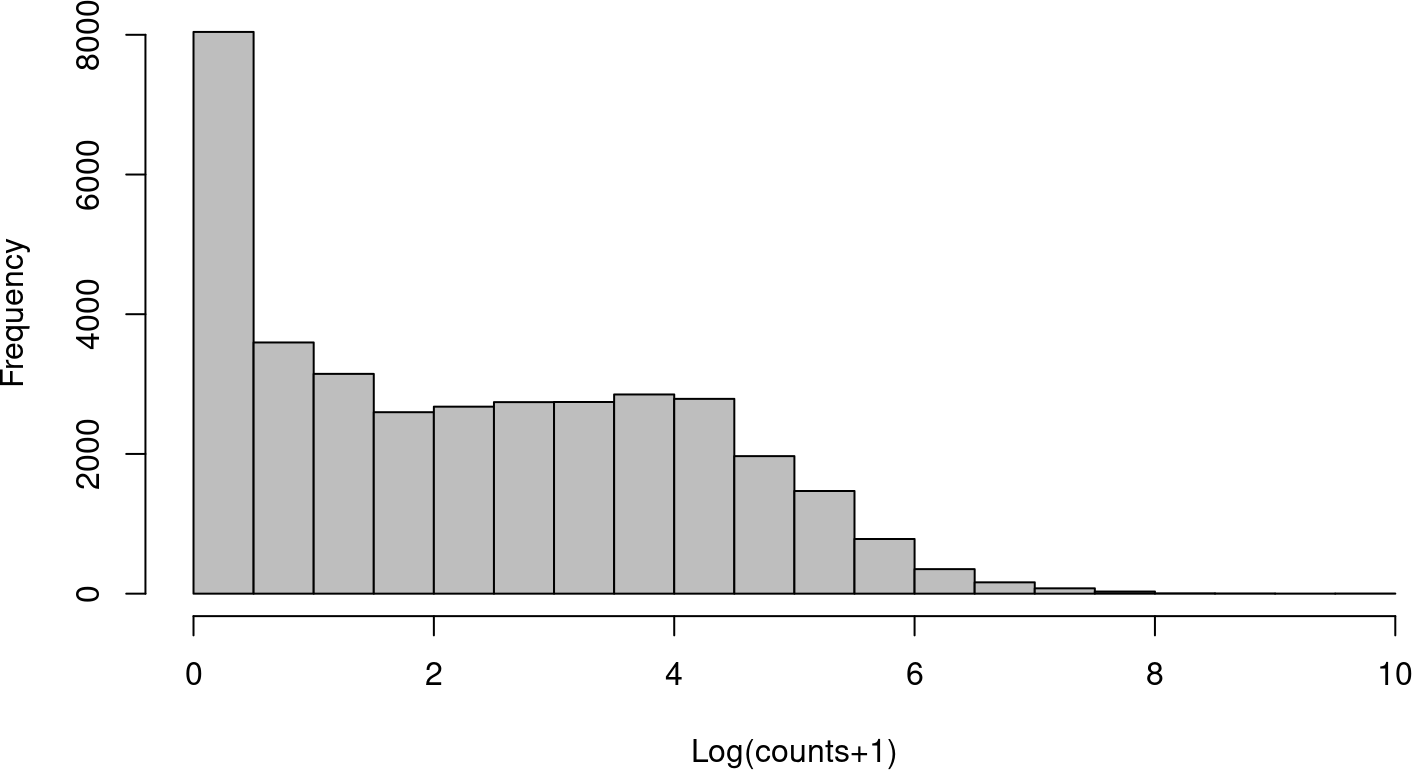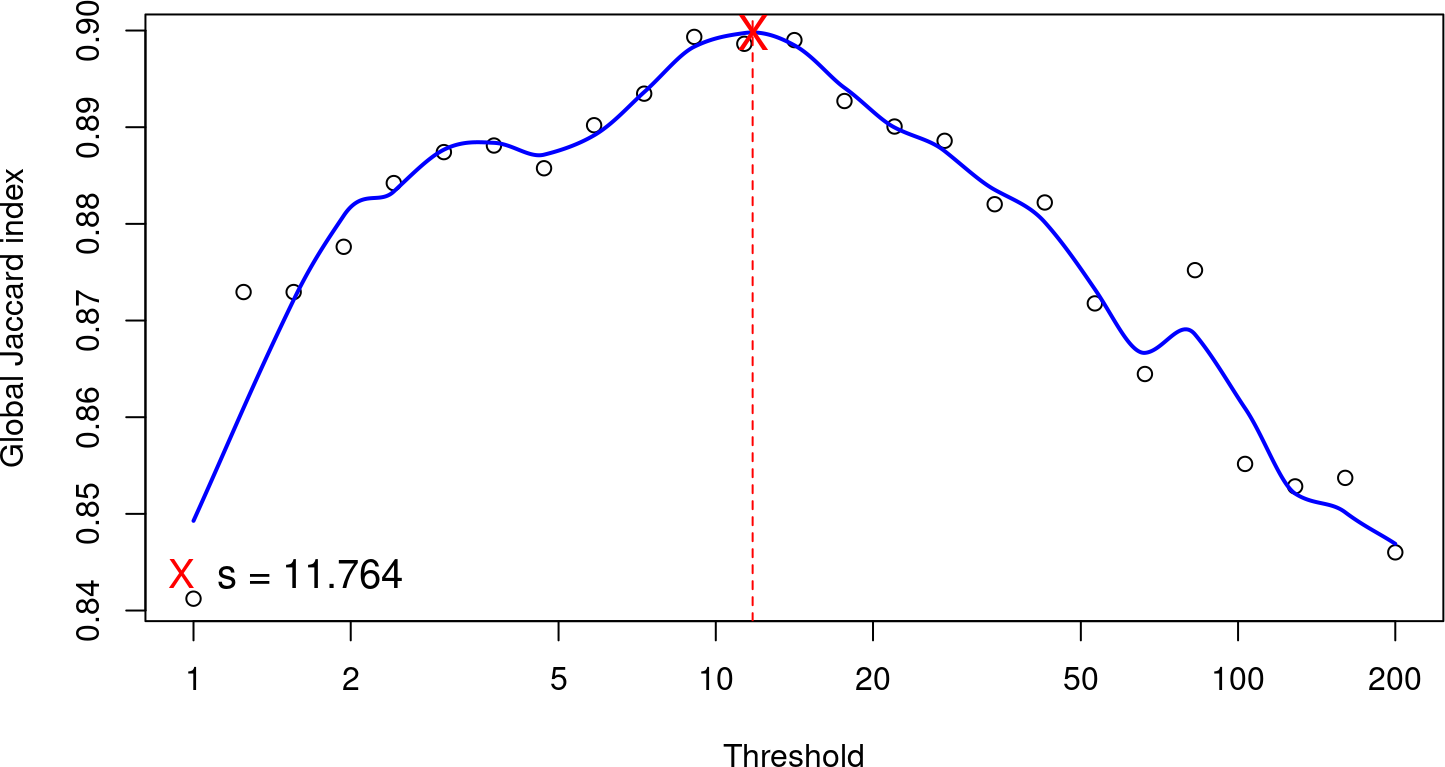This vignette illustrates the use of the HTSFilter package to filter replicated data from transcriptome sequencing experiments (e.g., RNA sequencing data) for a variety of different data classes: `matrix`, `data.frame`, the S3 classes associated with the edgeR package (`DGEExact` and `DGELRT`), and the S4 class associated with the DESeq2 package (`DESeqDataSet`).

# 1 Introduction

High-throughput sequencing (HTS) data, such as RNA-sequencing (RNA-seq) data, are increasingly used to conduct differential analyses, in which statistical tests are performed for each biological feature (e.g., a gene, transcript, exon) in order to identify those whose expression levels show systematic covariation with a particular condition, such as a treatment or phenotype of interest. For the remainder of this vignette, we will focus on gene-level differential analyses, although these methods may also be applied to differential analyses of (count-based measures of) transcript- or exon-level expression.

Because hypothesis tests are performed for gene-by-gene differential analyses, the obtained \(p\)-values must be adjusted to correct for multiple testing. However, procedures to adjust \(p\)-values to control the number of detected false positives often lead to a loss of power to detect truly differentially expressed (DE) genes due to the large number of hypothesis tests performed. To reduce the impact of such procedures, independent data filters are often used to identify and remove genes that appear to generate an uninformative signal (Bourgon, Gentleman, and Huber 2010); this in turn moderates the correction needed to adjust for multiple testing. For independent filtering methods for microarray data, see for example the genefilter Bioconductor package (Gentleman et al. 2020).

The HTSFilter package implements a novel data-based filtering procedure based on the calculation of a similarity index among biological replicates for read counts arising from replicated transcriptome sequencing (RNA-seq) data. This technique provides an intuitive data-driven way to filter high-throughput transcriptome sequencing data and to effectively remove genes with low, constant expression levels without incorrectly removing those that would otherwise have been identified as DE. The two fundamental assumptions of the filter implemented in the HTSFilter package are as follows:

• Biological replicates are present for each experimental condition, and
• Data can be appropriately normalized (scaled) to correct for systematic inter-sample biases.

Assuming these conditions hold, HTSFilter implements a method to identify a filtering threshold that maximizes the filtering similarity among replicates, that is, one where most genes tend to either have normalized counts less than or equal to the cutoff in all samples (i.e., filtered genes) or greater than the cutoff in all samples (i.e., non-filtered genes). This filtering similarity is defined using the global Jaccard index, that is, the average Jaccard index calculated between pairs of replicates within each experimental condition; see Rau et al. (2013) for more details.

For more information about between-sample normalization strategies, see Dillies et al. (2013); in particular, strategies for normalizing data with differences in library size and composition may be found in Anders and Huber (2010) and Robinson and Oshlack (2010), and strategies for normalizing data exhibiting sample-specific biases due to GC content may be found in Risso et al. (2011) and Hansen, Irizarry, and Wu (2012). Within the HTSFilter package, the Trimmed Means of M-values (TMM) (Robinson and Oshlack 2010) and DESeq (Anders and Huber 2010) normalization strategies may be used prior to calculating an appropriate data-based filter. If an alternative normalization strategy is needed or desired, the normalization may be applied prior to filtering the data with `normalization="none"`in the `HTSFilter` function; see Section 4 for an example.

The HTSFilter package is able to accommodate unnormalized or normalized replicated count data in the form of a `matrix` or `data.frame` (in which each row corresponds to a biological feature and each column to a biological sample), one of the S3 classes associated with the edgeR package (`DGEList`, `DGEExact`, `DGEGLM`, and `DGELRT`), or `DESeqDataSet` (the S4 class associated with the DESeq2 package), as illustrated in the following sections.

Finally, we note that the filtering method implemented in the HTSFilter package is designed to filter transcriptome sequencing, and not microarray, data; in particular, the proposed filter is effective for data with features that take on values over a large order of magnitude and with a subset of features exhibiting small levels of expression across samples (see, for example, Figure 1). In this vignette, we illustrate its use on count-based measures of gene expression, although its use is not strictly limited to discrete data.

# 2 Input data

For the purposes of this vignette, we make use of data from a study of sex-specific expression of liver cells in human and the DESeq2 and edgeR packages for differential analysis. Sultan et al. (2008) obtained a high-throughput sequencing data (using a 1G Illumina Genome Analyzer sequencing machine) from a human embryonic kidney and a B cell line, with two biological replicates each. The raw read counts and phenotype tables were obtained from the ReCount online resource (Frazee, Langmead, and Leek 2011).

To begin, we load the HTSFilter package, attach the gene-level count data contained in `sultan`, and take a quick look at a histrogram of gene counts:

``````library(HTSFilter)
library(edgeR)
library(DESeq2)
data("sultan")
hist(log(exprs(sultan)+1), col="grey", breaks=25, main="",
xlab="Log(counts+1)")``````Figure 1: Histogram of log transformed counts from the Sultan data
This illustrates the large number of genes with very small counts as well as the large heterogeneity in counts observed.

``pData(sultan)``
``````##           sample.id num.tech.reps    cell.line
## SRX008333 SRX008333             1 Ramos B cell
## SRX008334 SRX008334             1 Ramos B cell
## SRX008331 SRX008331             1      HEK293T
## SRX008332 SRX008332             1      HEK293T``````
``dim(sultan)``
``````## Features  Samples
##     9010        4``````

The unfiltered data contain 9010 genes in four samples (two replicates per condition).

# 3 Use of HTSFilter with varying data types

## 3.1`matrix` and `data.frame` classes

To filter high-throughput sequencing data in the form of a `matrix` or `data.frame`, we first access the expression data, contained in `exprs(sultan)`, and create a vector identifying the condition labels for each of the samples via the `pData` Biobase function. We then filter the data using the `HTSFilter` function, specifying that the number of tested thresholds be only 25 (`s.len=25`) rather than the default value of 100 to reduce computation time for this example. Note that as it is unspecified, the default normalization method is used for filtering the data, namely the Trimmed Mean of M-values (TMM) method of Robinson and Oshlack (2010). To use the DESeq normalization method (Anders and Huber 2010) `normalization="DESeq"` may be specified.

``````mat <- exprs(sultan)
conds <- as.character(pData(sultan)\$cell.line)

## Only 25 tested thresholds to reduce computation time
filter <- HTSFilter(mat, conds, s.min=1, s.max=200, s.len=25)``````Figure 2: Global Jaccard index for the Sultan data
The index is calculated for a variety of threshold values after TMM normalization, with a loess curve (blue line) superposed and data-based threshold values (red cross and red dotted line) equal to 11.764.

``````mat <- filter\$filteredData
dim(mat)``````
``##  4995    4``
``dim(filter\$removedData)``
``##  4015    4``

For this example, we find a data-based threshold equal to 11.764; genes with normalized values less than this threshold in all samples are filtered from subsequent analyses. The proposed filter thus removes 4015 genes from further analyses, leaving 4995 genes.

We note that an important part of the filter proposed in the HTSFilter package is a check of the behavior of the global similarity index calculated over a range of threshold values, and in particular, to verify that a reasonable maximum value is reached for the global similarity index over the range of tested threshold values (see Figure 2); the maximum possible value for the global Jaccard index is nearly 1. To illustrate the importance of this check, we attempt to re-apply the proposed filter to the previously filtered data (in practice, of course, this would be nonsensical):

``````par(mfrow = c(1,2), mar = c(4,4,2,2))
filter.2 <- HTSFilter(mat, conds, s.len=25)
dim(filter.2\$removedData)``````
``##  0 4``
``````hist(log(filter.2\$filteredData+1), col="grey", breaks=25, main="",
xlab="Log(counts+1)")``````